### ATLAS_2014_I1326641

Back to index

Double-differential three-jet production cross-sections have been measured in proton-proton collisions at a centre-of-mass energy of $\sqrt{s} = 7\,$TeV using the ATLAS detector at the Large Hadron Collider. The measurements are presented as a function of the three-jet mass ($m_{jjj}$), in bins of the sum of the absolute rapidity separations between the three leading jets ($\left|Y^\ast\right|$). Invariant masses extending up to 5\,TeV are reached for $8<\left|Y^\ast\right|<10$. These measurements use a sample of data recorded using the ATLAS detector in 2011, which corresponds to an integrated luminosity of $4.51\,\text{fb}^{-1}$. Jets are identified using the anti-$k_\text{t}$ algorithm with two different jet radius parameters, $R=0.4$ and $R=0.6$.

ATLAS_2014_I1326641_d01-x01-y01: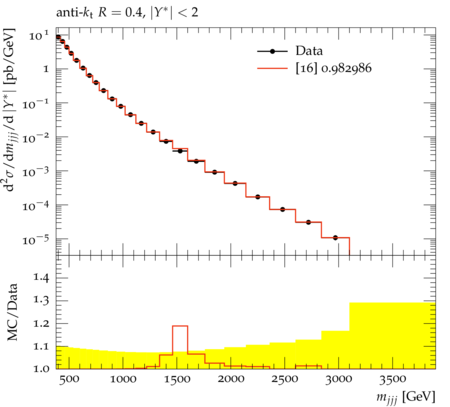ATLAS_2014_I1326641_d02-x01-y01: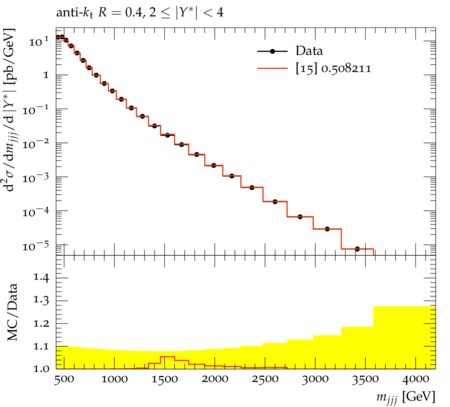ATLAS_2014_I1326641_d03-x01-y01: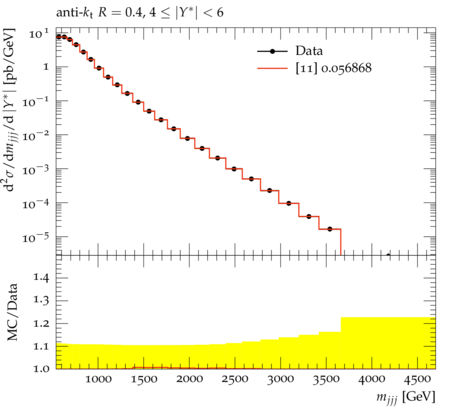ATLAS_2014_I1326641_d04-x01-y01: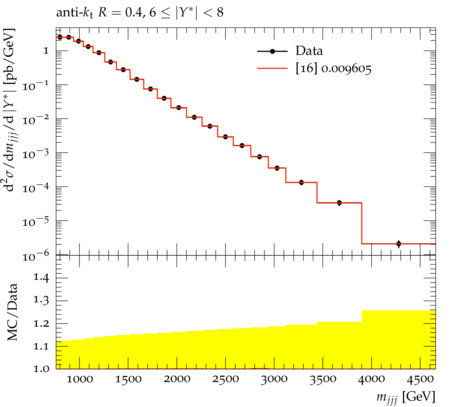ATLAS_2014_I1326641_d05-x01-y01: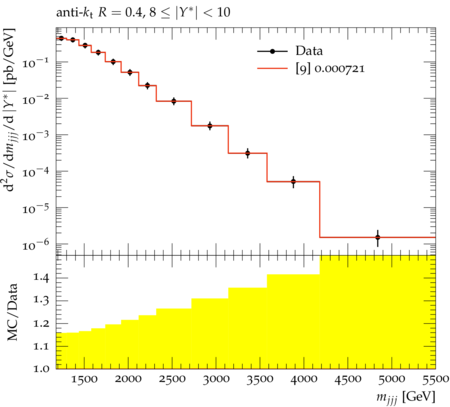ATLAS_2014_I1326641_d06-x01-y01: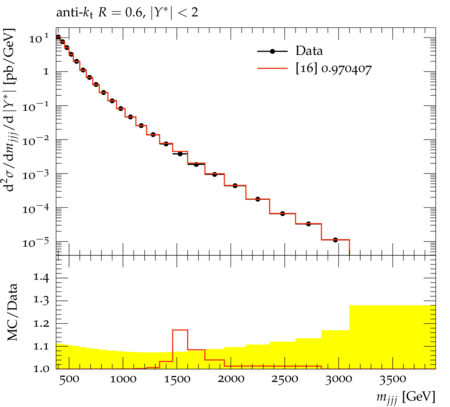ATLAS_2014_I1326641_d07-x01-y01: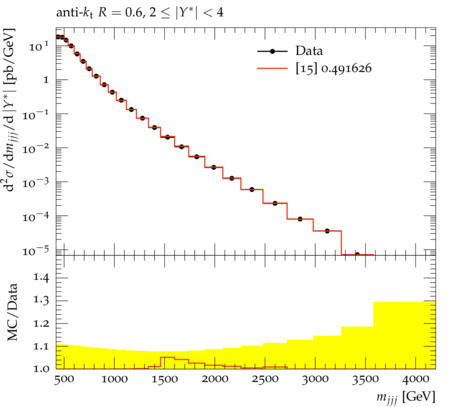ATLAS_2014_I1326641_d08-x01-y01: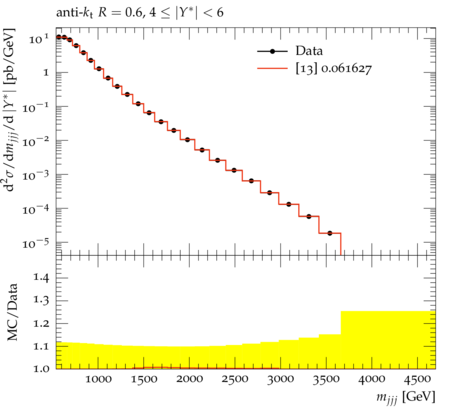ATLAS_2014_I1326641_d09-x01-y01: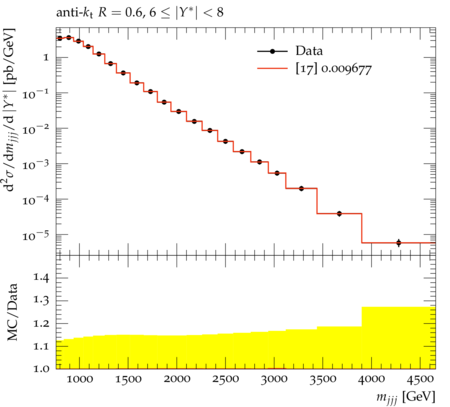ATLAS_2014_I1326641_d10-x01-y01: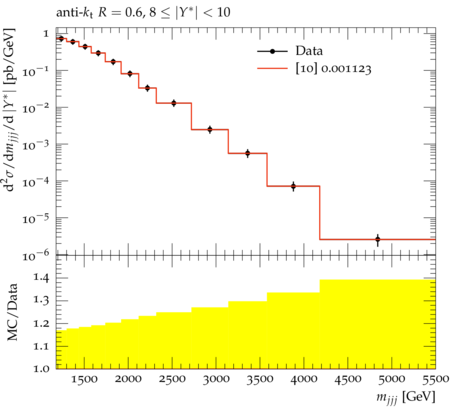Generated at Saturday, 23. September 2017 11:56AM